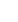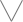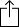Feedback
C
D
E
F
G
A
B
1
2
3
4
5

1
2
3
4
5
6
7
8
9
10
11
12
13
14
15
16
17
18
19
20
21
22
23
24
E
B
G
D
A
E
F
F
G
A
A
A
B
C
C
D
D
E
F
F
G
A
A
A
B
C
C
D
D
E
C
C
D
D
E
F
F
G
A
A
A
B
C
C
D
D
E
F
F
G
A
A
A
B
A
A
A
B
C
C
D
D
E
F
F
G
A
A
A
B
C
C
D
D
E
F
F
G
D
E
F
F
G
A
A
A
B
C
C
D
D
E
F
F
G
A
A
A
B
C
C
D
A
B
C
C
D
D
E
F
F
G
A
A
A
B
C
C
D
D
E
F
F
G
A
A
F
F
G
A
A
A
B
C
C
D
D
E
F
F
G
A
A
A
B
C
C
D
D
E

# B Major Eleventh for Guitar - CAGED Chart

A B Major eleventh chord, also written as Bmaj11, BΔ11, Bma11 or BM11 contains the notes B, D#, F#, A#, C# and E. The chord is comprised of the intervals M3, m3, M3, m3 and m3.### Scale Notes

B
D
F
A
C
EM3
m3
M3
m3
m3
• Options
• Playerand then